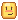# I made a rolling dice script! totally random

``````local dice2 = game.Workspace.Dice2

local d1_pos_rand_vector3_X = math.random(20, 24)
local d1_pos_rand_vector3_Y = math.random(20, 24)
local d1_pos_rand_vector3_Z = math.random(-5, -3)

local d1_rot_rand_vector3_X = math.random(-180, 180)
local d1_rot_rand_vector3_Y = math.random(-180, 180)
local d1_rot_rand_vector3_Z = math.random(-180, 180)

local d2_pos_rand_vector3_X = math.random(20, 24)
local d2_pos_rand_vector3_Y = math.random(20, 24)
local d2_pos_rand_vector3_Z = math.random(3, 5)

local d2_rot_rand_vector3_X = math.random(-180, 180)
local d2_rot_rand_vector3_Y = math.random(-180, 180)
local d2_rot_rand_vector3_Z = math.random(-180, 180)

dice1.Position = Vector3.new(d1_pos_rand_vector3_X, d1_pos_rand_vector3_Y, d1_pos_rand_vector3_Z)
dice1.Orientation = Vector3.new(d1_rot_rand_vector3_X, d1_rot_rand_vector3_Y, d1_rot_rand_vector3_Z)

dice2.Position = Vector3.new(d2_pos_rand_vector3_X, d2_pos_rand_vector3_Y, d2_pos_rand_vector3_Z)
dice2.Orientation = Vector3.new(d2_rot_rand_vector3_X, d2_rot_rand_vector3_Y, d2_rot_rand_vector3_Z)

wait(8)

dice1.Anchored = false
dice2.Anchored = false
``````

rolling dice script

5 Likes

is there anything wrong with this lol i have made scripts in the past and had people point out things which seemed insignificant to me

2 Likes

I’d recommend you to test it out as, if it doesn’t work we know something doesn’t work.

However, I believe someone might steal the script if you do not test it. If you test it and it works, I would not put the script. If it fails, no one will steal it.

Keep in mind, I’m not a scripter. Looks interesting though!thanks for the feedback, ill keep that i mind and delete it some time today3 Likes

I’d avoid advertising it as “totally random” - there’s no such thing in computers, though if one did invent it then it would definitely be a cool creation.

Using the pseudorandom function math.random, if given the same seed, the same pattern of numbers would be generated.

2 Likes

Oh, I didn’t know that, guess I learnt something new today… So is it sort of an illusion of randomness?

1 Like

Yeah exactly, it’s very hard to predict what the next number will be, but ultimately the computer is just putting a seed number through a complicated algorithm.

If you set `math.randomseed( X )` to the same value each time the game plays, for example, you will get the exact same sequence of generated numbers. Sometimes this is actually useful, but most of the time people set it to a number that changes (e.g. the current timestamp when the game starts) to ensure variety. But don’t worry, this is similar to what happens internally so you don’t need to manually set it if you want the behaviour.From: math

Pseudorandom is sort of close enough to random that you can’t tell the difference for a given sequence of generated numbers, but it’s just worth noting when describing code to others2 Likes

Hmm… complicated, but I get the gist.1 Like